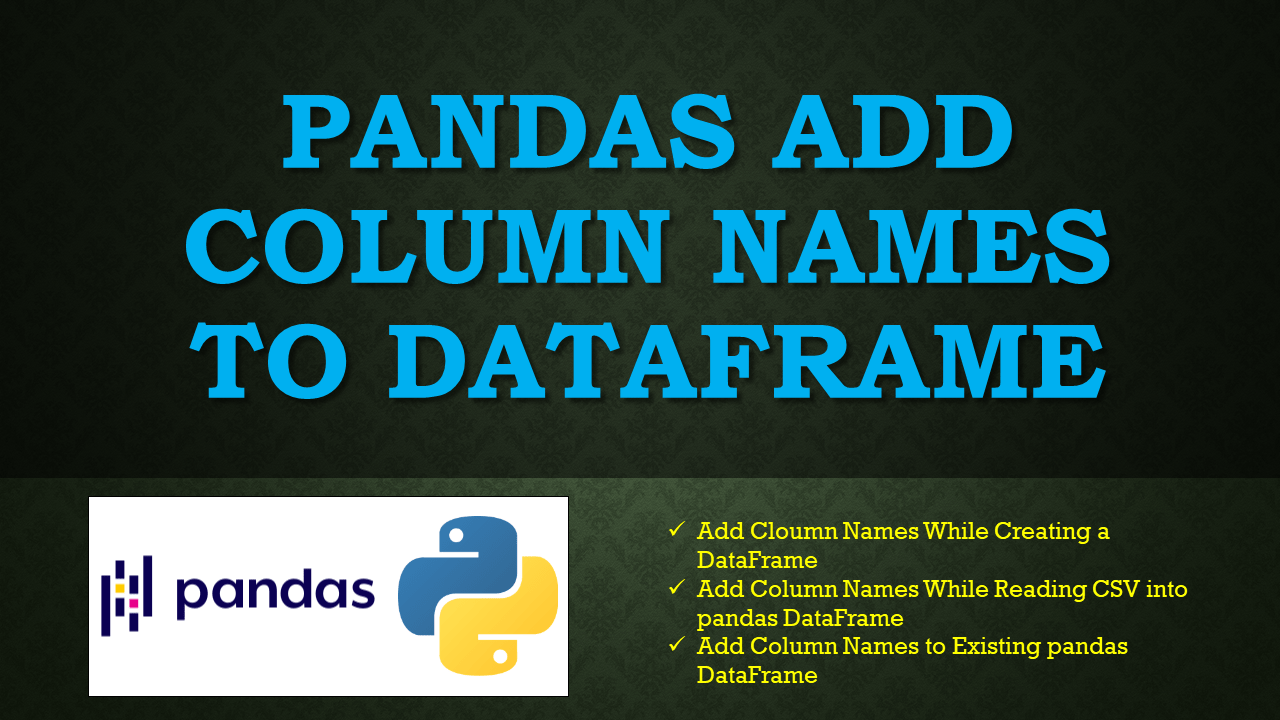# Pandas Add Column Names to DataFrame

• Post author:
• Post category:Pandas

You can add column names to pandas at the time of creating DataFrame or assign them after creating. Sometimes you might receive a CSV file that doesn’t have names and you would need to add after reading CSV data into DataFrame.

In this article, I will explain how to add/set/assign column names to DataFrame with several examples. The column names on DataFrame are used to identify what type of data each column holds.

## 1. Quick Examples of pandas Add Column Names

Below are some quick examples of how to add/assign or set column labels to DataFrame.

``````
# Below are quick examples

# Column names to be added
column_names=["Courses","Fee",'Duration']

# Create DataFrame by assigning column names
df=pd.DataFrame(technologies, columns=column_names)

# Add column names to existing DataFrame
df.columns = column_names
``````

## 2. Add Cloumn Names to DataFrame

You can add column names to pandas DataFrame while creating manually from the data object. In order to create a DataFrame, you would use a DataFrame constructor which takes a `columns` param to assign the names. It takes a list as a value and the number of values in a list should not exceed the number of columns in DataFrame.

``````
# Import pandas Library
import pandas as pd
technologies = [ ["Spark",20000, "30days"],
["Pandas",25000, "40days"],
]

# Column names to be added
column_names=["Courses","Fee",'Duration']

# Create DataFrame by assigning column names
df=pd.DataFrame(technologies, columns=column_names)
print(df)
``````

Yields below output.

``````
# Output:
Courses    Fee Duration
0   Spark  20000   30days
1  Pandas  25000   40days
``````

pandas read_csv() method has an option to identify the column names that are presented in a CSV file, In case your CSV file doesn’t have on the first row then you can add custom names while reading a CSV into pandas DataFrame.

``````
# Column names to be added
column_names=["Courses","Fee",'Duration']

``````

## 3. Add Column Names to Existing DataFrame.

In the above sections, you have seen how to add while creating a DataFrame. Sometimes it’s not possible to know the column names up-front and you may need to add names to the existing DataFrame.

``````
import pandas as pd

# Create DataFrame with out column names
df=pd.DataFrame([ ["Spark",20000, "30days"],
["Pandas",25000, "40days"],
])

# Assign column names to Existing DataFrame
column_names=["Courses","Fee",'Duration']
df.columns = column_names
print(df)
``````

Yields same output as above.

## 4. Complete Examples

Below is the complete example of how to assign column names to DataFram.

``````
# Import pandas Library
import pandas as pd
technologies = [ ["Spark",20000, "30days"],
["Pandas",25000, "40days"],
]

# Column names to be added
column_names=["Courses","Fee",'Duration']

# Create DataFrame by assigning column names
df=pd.DataFrame(technologies, columns=column_names)
print(df)

# Create DataFrame with out column labels
df=pd.DataFrame([ ["Spark",20000, "30days"],
["Pandas",25000, "40days"],
])

# Assign column names to existing DataFrame
column_names=["Courses","Fee",'Duration']
df.columns = column_names
print(df)
``````

## Conclusion

In this article, you have learned to assign column names to pandas DataFrame, while creating, when reading a CSV and to an existing DataFrame.

### Naveen

I am a Data Engineer with 20+ years of experience in transforming data into actionable insights. Over the years, I have honed my expertise in designing, implementing, and maintaining data pipelines with frameworks like Apache Spark, PySpark, Pandas, R, Hive and Machine Learning. My journey in the field of data engineering has been a continuous learning, innovation, and a strong commitment to data integrity. I have started this SparkByExamples.com to share my experiences with the data as I come across. You can learn more about me at LinkedIn